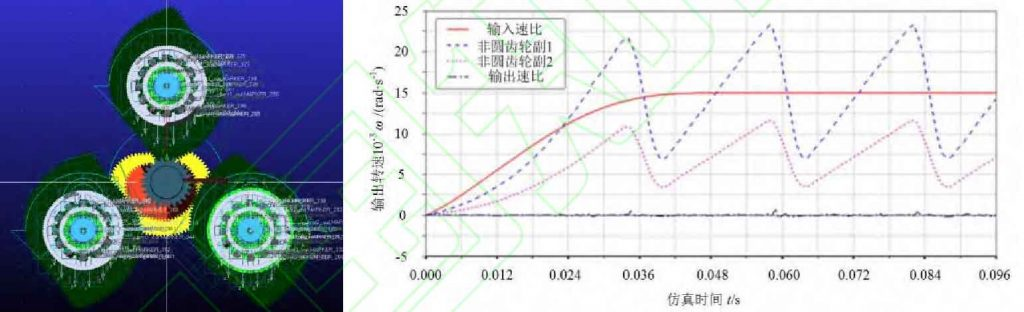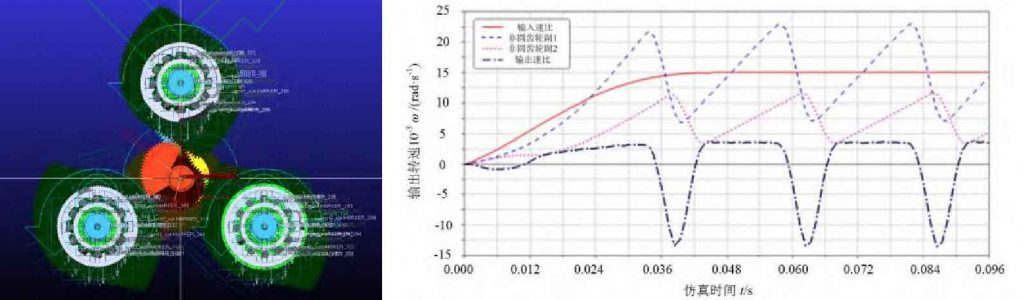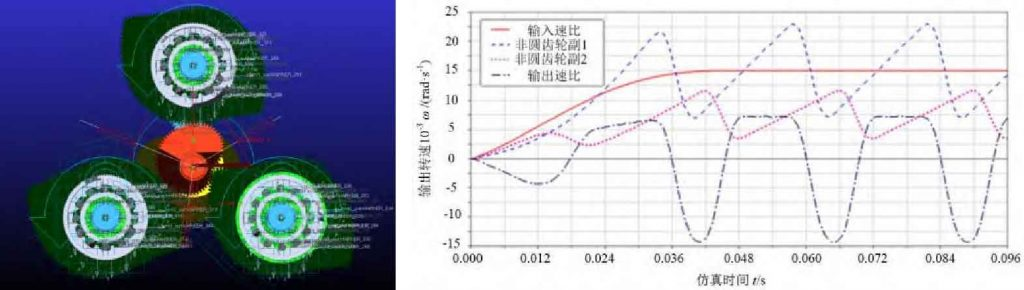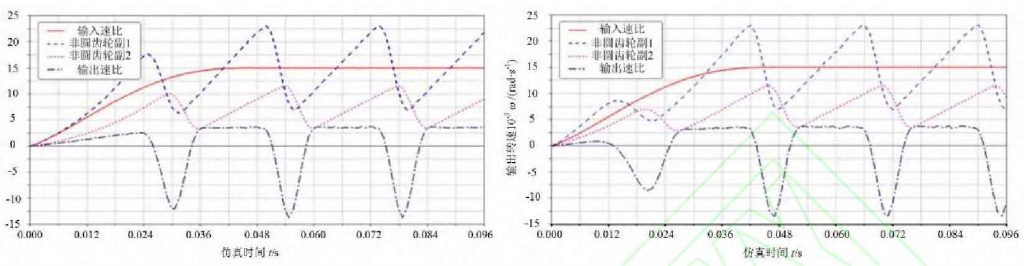# Simulation verification of noncircular gear stepless speed regulation

## 1. Modeling of non-circular gear stepless speed regulation mechanism

This design mainly lies in the research of the output speed ratio law of the main part of the non-circular gear stepless speed regulation mechanism, so it mainly includes the driving shaft for power input, the non-circular gear pair for time variable ratio production, the differential gear train for speed ratio integration and the parallel shaft gear pair as the output selection part, as shown in Figure 1.

The model shown in Figure 1 is imported into Adams (automatic dynamic analysis), and the corresponding motion pair is added. The input shaft is used as the power input. After the speed ratio generation of non-circular gear and the speed ratio integration of differential gear train, the required time-varying function is obtained. Finally, the parallel shaft gear train selects the corresponding working section branch for time-varying output (see Figure 2).

## 2. Simulation analysis

In order to verify the stability and variability of the output speed ratio of the non-circular gear stepless speed regulation mechanism, it is necessary to simulate the output speed ratio of the non-circular gear stepless speed regulation mechanism with different phase differences. The input speed is 262 radgs, and the load torque at the output end is 200 N · mm; The simulation time is 0.1 s; The simulation output speed ratio characteristics of stepless speed regulation mechanism under different phase differences are obtained, as shown in Fig. 3 ~ Fig. 5.(a) Positions of gears 1 and 2 of non-circular gear pair(b) Time varying output speed ratio characteristic curve of each part of stepless speed regulation mechanism(a) Positions of gears 1 and 2 of non-circular gear pair(b) Time varying output speed ratio characteristic curve of each part of stepless speed regulation mechanism(a) Positions of gears 1 and 2 of non-circular gear pair(b) Output speed ratio characteristic curve of the first branch of stepless speed regulation mechanism(c) Output speed ratio characteristic curve of the second branch of stepless speed regulation mechanism(d) Output speed ratio characteristic curve of the third branch of stepless speed regulation mechanism

Compared with the simulation results of non-circular gear stepless speed regulation mechanism under different phase angles shown in Fig. 3-5, when the phase difference of non-circular gear pair 1 and 2 is constant, non-circular gear pair 1 and 2 can achieve the preset first-order speed ratio function, and the stable output speed ratio corresponding to the working section angle is obtained through the speed ratio integration part; Further comparison with FIG. 5 (b) – (d) shows that the stable output speed ratio functions of different non-circular gear branches have different phase differences, and continuous and stable time-varying output can be realized through the output selection part.